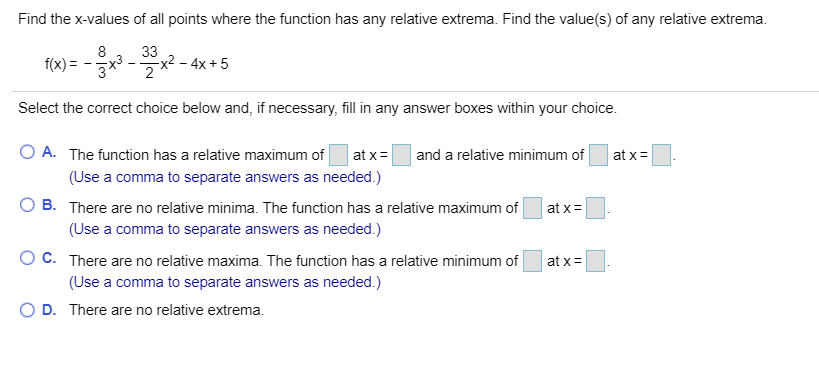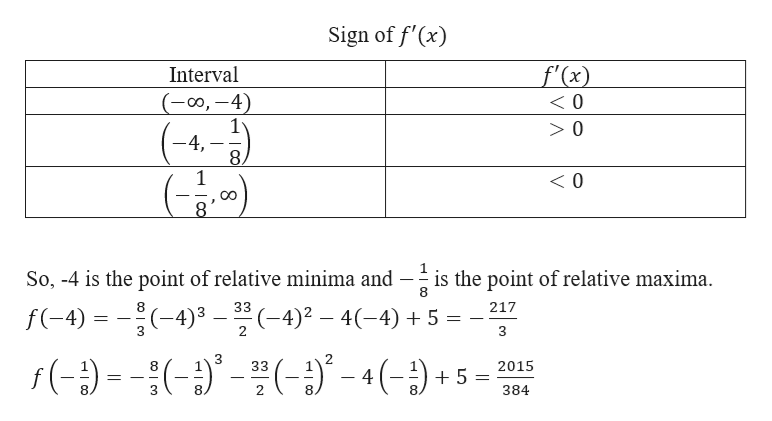# Find the x-values of all points where the function has any relative extrema. Find the value(s) of any relative extrema33x2 - 4x +52f(x)Select the correct choice below and, if necessary, fill in any answer boxes within your choiceO A. The function has a relative maximum ofat xand a relative minimum ofat x(Use a comma to separate answers as needed.)B. There are no relative minima. The function has a relative maximum ofat x(Use a comma to separate answers as needed.)O C. There are no relative maxima. The function has a relative minimum ofat x(Use a comma to separate answers as needed.)D. There are no relative extrema.

Questionhelp_outlineImage TranscriptioncloseFind the x-values of all points where the function has any relative extrema. Find the value(s) of any relative extrema 33 x2 - 4x +5 2 f(x) Select the correct choice below and, if necessary, fill in any answer boxes within your choice O A. The function has a relative maximum of at x and a relative minimum of at x (Use a comma to separate answers as needed.) B. There are no relative minima. The function has a relative maximum of at x (Use a comma to separate answers as needed.) O C. There are no relative maxima. The function has a relative minimum of at x (Use a comma to separate answers as needed.) D. There are no relative extrema. fullscreen
check_circle

Step 1

Given,

Step 2

Now, differentiating with respect to x, we get

Step 3

A relative maximum point is a point where the function changes direction from increasing to decreasing.

Similarly, ...help_outlineImage TranscriptioncloseSign of f'(x) f'(x) Interval (00,-4) 0 -4 8 1 < 0 So, -4 is the point of relative minima and --- is the point of relative maxima. f(-4) -4)3(-4)4-4) 8 217 ( -(- f () 8 33 2015 4 2. 384 fullscreen

### Want to see the full answer?

See Solution

#### Want to see this answer and more?

Solutions are written by subject experts who are available 24/7. Questions are typically answered within 1 hour.*

See Solution
*Response times may vary by subject and question.
Tagged in

### Derivative